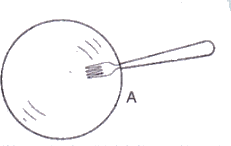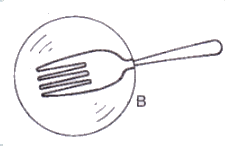# Solutions of Refraction of Light (Page No- 253 & 255) - Physics By Lakhmir Singh, Class 10 Class 10 Notes | EduRev

## Class 10 : Solutions of Refraction of Light (Page No- 253 & 255) - Physics By Lakhmir Singh, Class 10 Class 10 Notes | EduRev

The document Solutions of Refraction of Light (Page No- 253 & 255) - Physics By Lakhmir Singh, Class 10 Class 10 Notes | EduRev is a part of the Class 10 Course Class 10 Physics Solutions By Lakhmir Singh & Manjit Kaur.
All you need of Class 10 at this link: Class 10

Lakhmir Singh Physics Class 10 Solutions Page No:253

Question 23: When an object is placed 10 cm in front of lens A, the image is real, inverted, magnified and formed at a great distance. When the same object is placed 10 cm in front of lens B, the image formed is real, inverted and same size as the object.
(a) What is the focal length of lens A ?
(b) What is the focal length of lens B ?
(c) What is the nature of lens A ?
(d) What is the nature of lens B ?

Solution : (a) The object is placed at focus, so f=10 cm.
(b) the object is placed at a distance twice the focallength, so f=5 cm.
(c) Convex lens (since image is real).
(d) Convex lens (since image is real).

Question 24: When a fork is seen through lenses A and B one by one, it appears as shown in the diagrams. What is the nature of (i) lens A, and (ii) lens B ? GSolution : (i) Concave lens because of negative magnification.
(ii) Convex lens because of positive magnification.

Question 25: What kind of lens can form :
(a) an inverted magnified image ?
(b) an erect magnified image ?
(c) an inverted diminished image ?
(d) an erect diminished image ?

Solution : (a) Convex lens.
(b) Convex lens.
(c) Convex lens.
(d) Concave lens.

Lakhmir Singh Physics Class 10 Solutions Page No:255

Question 1: The lens A produces a magnification of, – 0.6 whereas lens B produces a magnification of + 0.6.
(a) What is the nature of lens A ?
(b) What is the nature of lens B ?

Solution : (a) Convex lens (since image is real, inverted and diminished).
(b) Concave lens (since image is virtual, erect and diminished).

Question 2: A 50 cm tall object is at a very large distance from a diverging lens. A virtual, erect and diminished image of the object is formed at a distance of 20 cm in front of the lens. How much is the focal length of the lens ?

Solution : When an object is placed at a very large distance from a diverging lens, then image is formed at the focus of the lens.
Therefore, the focal length of the lens is 20 cm.

Offer running on EduRev: Apply code STAYHOME200 to get INR 200 off on our premium plan EduRev Infinity!

94 docs

,

,

,

,

,

,

,

,

,

,

,

,

,

,

,

,

,

,

,

,

,

,

,

,

;